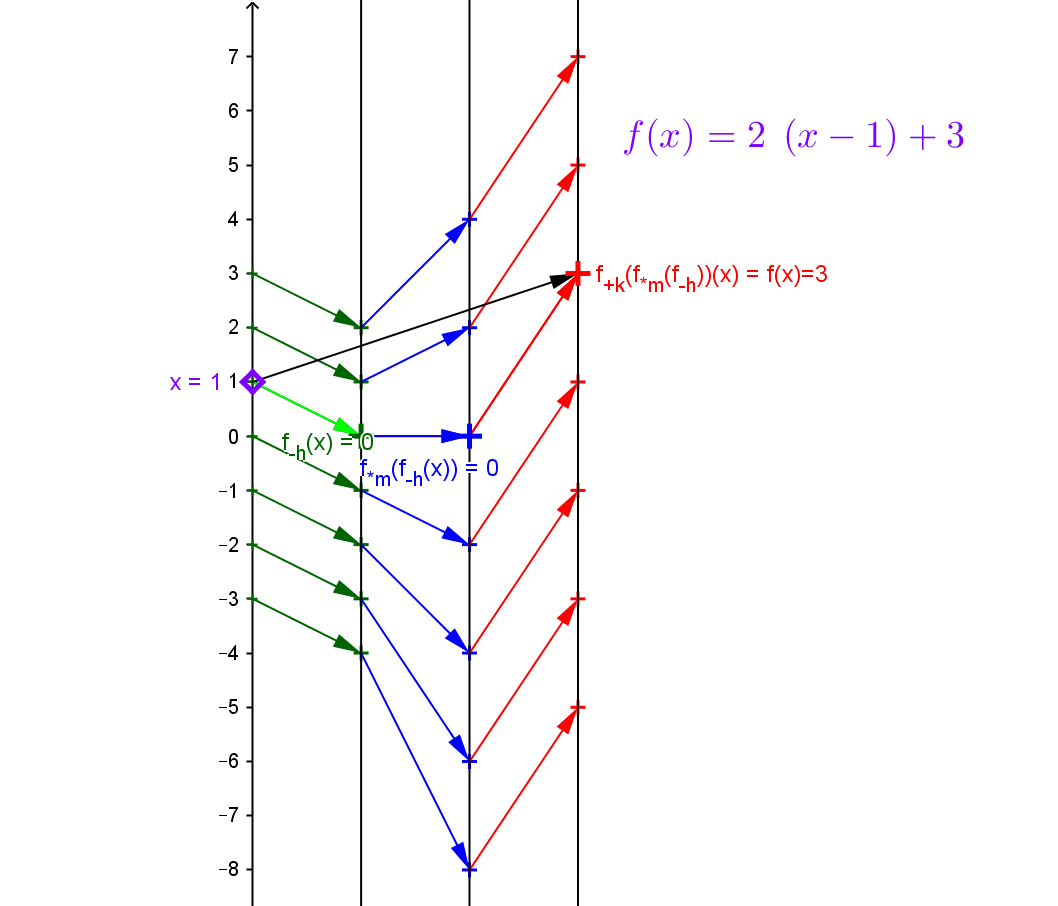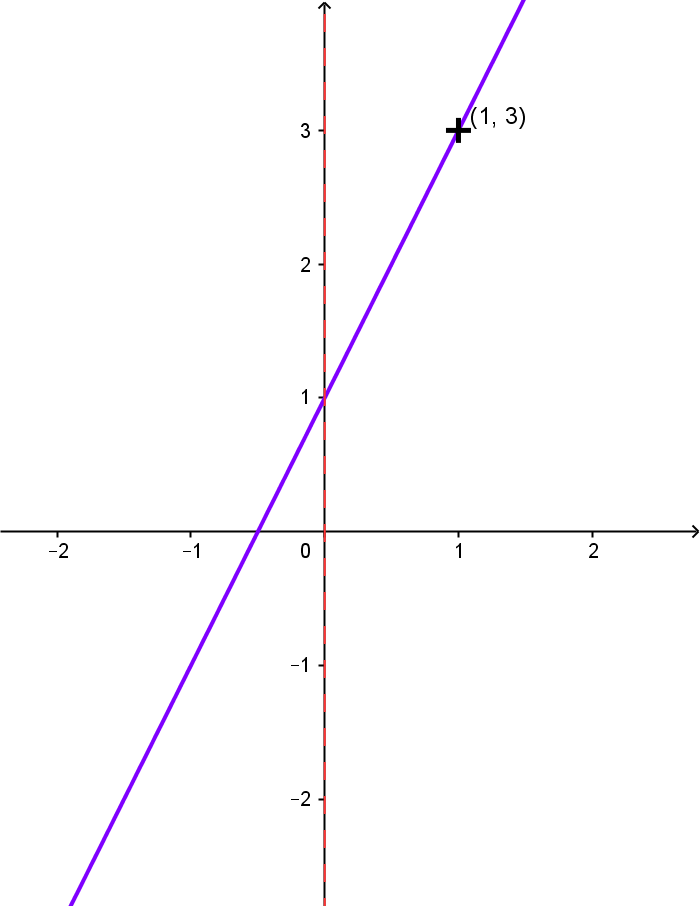Example LF.FORM.4 : Suppose $f$ is a linear function with slope $=m=2$ and $f(1) = 3$. Find the point-slope form of the line. Visualize $f$ with a mapping diagram that illustrates the function as the composition of the three core linear functions  $f_{+ 3}\circ f_{*2}\circ f_{ -1}$. Note the arrow for $f(1) =3$ and that based on $m=2$, $f(2)=5$ and $f(0)=1$ is visualized by the spacing of the arrows in the mapping diagram.

Solution: We use the point slope form with $m=2$ and $f(1)=3$ to obtain $f(x) = 3 + 2*(x-1).$

Draw a mapping diagram yourself or use the GeoGebra figure.
Given a point / number, $x$, on the source line, there is a unique arrow  meeting the target line at the point / number, $3 + 2*(x-1) = 2x + 1$, which corresponds to the linear function's value for $x$.When the point in the domain is $x = 1$, the black arrow points to $f(1) = 3$ visualizing the point $(1,3)$ on the graph of $f$. We also have $f_{+ 3} \circ f_{*2} \circ f_{ -1} (1) = f_{+3} \circ f_{*2}(0)= f_{+3}(0) = 3$ which is visualized in the mapping diagram for the composition by arrows $1 \rightarrow 0 \rightarrow 0 \rightarrow 3$ .

Since a unit step on the source axis is used in this mapping diagram, we see the slope (magnification, rate) visualized in the gap between the heads of consecutive arrows on the mapping diagram both in the middle section and the final arrows.
As  $m > 0$, the arrows never cross. Observe also that the function is increasing  at a rate of two units on the second and third target values for every unit increase in the domain value.